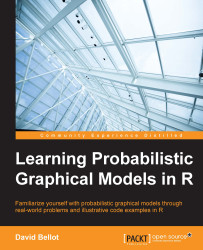•#### Learning Probabilistic Graphical Models in R#### Overview of this book

Probabilistic graphical models (PGM, also known as graphical models) are a marriage between probability theory and graph theory. Generally, PGMs use a graph-based representation. Two branches of graphical representations of distributions are commonly used, namely Bayesian networks and Markov networks. R has many packages to implement graphical models. We’ll start by showing you how to transform a classical statistical model into a modern PGM and then look at how to do exact inference in graphical models. Proceeding, we’ll introduce you to many modern R packages that will help you to perform inference on the models. We will then run a Bayesian linear regression and you’ll see the advantage of going probabilistic when you want to do prediction. Next, you’ll master using R packages and implementing its techniques. Finally, you’ll be presented with machine learning applications that have a direct impact in many fields. Here, we’ll cover clustering and the discovery of hidden information in big data, as well as two important methods, PCA and ICA, to reduce the size of big problems.
Learning Probabilistic Graphical Models in RCreditswww.PacktPub.comPrefaceFree Chapter
Probabilistic ReasoningExact InferenceLearning ParametersBayesian Modeling – Basic ModelsApproximate InferenceBayesian Modeling – Linear ModelsProbabilistic Mixture ModelsAppendixIndex## MCMC for probabilistic graphical models in R

In fact, this section could be the title of book. As a matter of fact, there are several books entirely devoted to this specific topic. Research in this field is extremely active, with many new algorithms coming every year.

There are numerous packages implementing MCMC algorithms for different types of algorithms. There are also more generic frameworks such as the famous BUGS (and its open source implementation OpenBUGS) and a new, even more powerful framework called Stan. Historically, BUGS was the first framework to popularize MCMC inference in Bayesian statistics and literally led to a revolution in this field, as everyone could benefit from Bayesian statistics right out of the box.

Making an introduction to each of them and showing all the possibilities of MCMC for a few specific graphical models would require another book as big as this one. In this section, we will therefore focus on a programming environment available in R. In fact, it works...